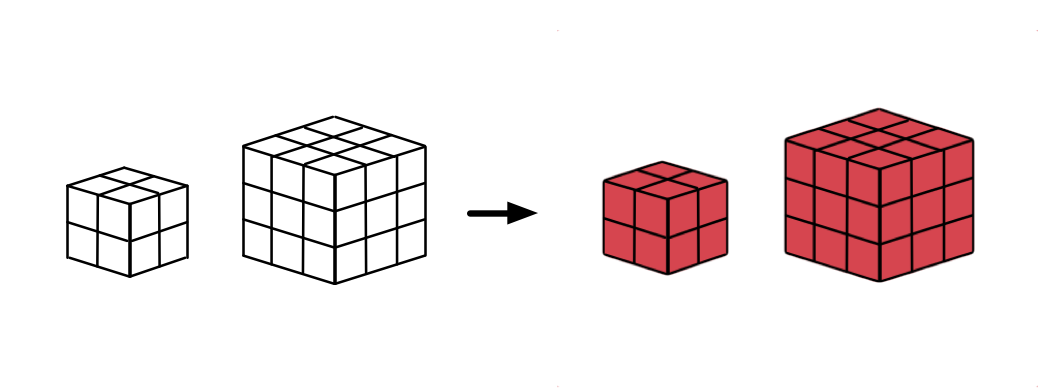# Unit Cubes 1 – Counting Faces

Probability Level 2Consider the outside of cubes made out of $1 \times 1 \times 1$ unit blocks, with side lengths $n \times n \times n$, for $n \geq 2$, which we have painted red on the outside.

The following table shows the number of unit cubes which are colored on a given number of faces.

 Cube Size 3 faces red 2 faces red 1 face red $2 \times 2 \times 2$ 8 0 0 $3 \times 3 \times 3$ 8 12 6 $4 \times 4 \times 4$ ? ? ?

What is the sum of the numbers missing from the row for the $4 \times 4 \times 4$ cube?

×

Problem Loading...

Note Loading...

Set Loading...